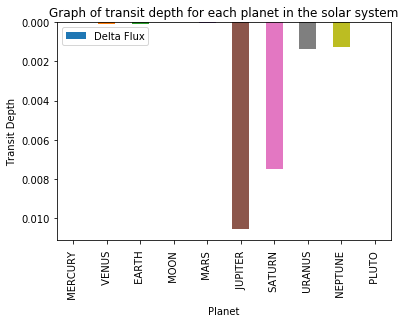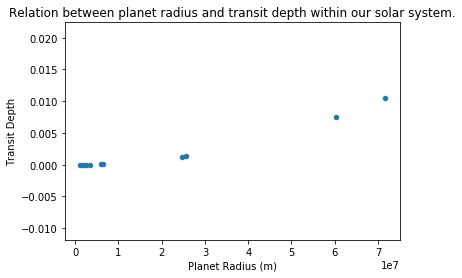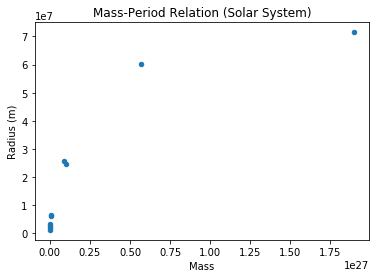# Exoplanets In My Solar System¶

### This notebook is based on the November 2019 transit of Mercury.¶

Planetary transits in the solar system are perfect analogies of explanetary transits. If sufficiently intelligent aliens were monitoring the brightness of our star on Monday 11th November 2019, they may have detected something strange.The above image is a simplified diagram of how planetary transits can be detected by measuring the dip in brightness of the host star, known as the "Transit Method". Below is an image I captured of the transit on the 11th. Note the tiny black dot. Thats Mercury!The change in brightness $\Delta F$ is related to the size of the planet $R_P$ through a very simple formula.

$\Delta F / F = (R_P/R_*)^2$

Lets now import some python libraries and get Juypter to do the calculation for us.

In :
import astropy.units as u
F = 1370 # Stellar Flux
R_p = 2.53E6 # Radius of Mercury in m
R_s = 6.95E8 # Radius of the Sun in m

F"The radius of Mercury is {R_p}, and the radius of the Sun is {R_s}"

Out:
'The radius of Mercury is 2530000.0, and the radius of the Sun is 695000000.0'
In :
delta_f_f = ((R_p/R_s)**2)
F"The drop in flux due to the transit of Mercury is {delta_f_f}"

Out:
'The drop in flux due to the transit of Mercury is 1.3251695046840224e-05'

Using the BATMAN python code, it is possible to simulate what the Mercury transit lightcurve would look like observer from a large distance.

In :
import batman
import numpy as np
import matplotlib.pyplot as plt

params = batman.TransitParams()
params.t0 = 0.                       #time of inferior conjunction
params.per = 88.                     #orbital period
params.a = 5.7E7/R_s                 #semi-major axis (in units of stellar radii)
params.inc = 87.                     #orbital inclination (in degrees)
params.ecc = 0.                      #eccentricity
params.w = 90.                       #longitude of periastron (in degrees)
params.u = [0.1, 0.3]                #limb darkening coefficients [u1, u2]
params.limb_dark = "quadratic"       #limb darkening model

t = np.linspace(-30, 30, 100)

m = batman.TransitModel(params, t)    #initializes model
flux = m.light_curve(params)          #calculates light curve

plt.plot(t, flux)
plt.xlabel("Time from central transit")
plt.ylabel("Relative flux")
plt.show()

<matplotlib.figure.Figure at 0xf6188b0>

The purpose of code is to make life easier. In the sections above, I have defined the variables R_s and R_p but going forward I want to be able to convert planet radii in meters to solar radii easily, so I will create a small subroutine to do this.

In :
def cvnt_sol(radius):

In :
example = cvnt_sol(6.05E6)
F"In this example I have converted the radius of Venus into solar radii. The result is {example}"

Out:
'In this example I have converted the radius of Venus into solar radii. The result is 0.008705035971223022'

Lets now use PANDAS to do some data manipulation.

In :
import pandas as pd # Import the PANDAS library

In :
file = "solar_system_data_1.csv" # Assign the data file to a variable.

In :
df = pd.read_csv(file) # Read in the data file into a dataframe.

In :
df # Display the dataframe.

Out:
Planet Mass(10^24kg) Radius(km) Diameter(km) Density (kg/m3) Gravity (m/s2) Escape Velocity (km/s) Rotation Period (hours) Length of Day (hours) Distance from Sun (106 km) ... Orbital Period (days) Orbital Velocity (km/s) Orbital Inclination (degrees) Orbital Eccentricity Obliquity to Orbit (degrees) Mean Temperature (C) Surface Pressure (bars) Number of Moons Ring System? Global Magnetic Field?
0 MERCURY 0.3300 2439.5 4879 5427 3.7 4.3 1407.6 4222.6 57.900 ... 88 47.4 7.0 0.205 0.034 167 0 0 No Yes
1 VENUS 4.8700 6052.0 12,104 5243 8.9 10.4 -5832.5 2802.0 108.200 ... 224.7 35.0 3.4 0.007 177.400 464 92 0 No No
2 EARTH 5.9700 6378.0 12,756 5514 9.8 11.2 23.9 24.0 149.600 ... 365.2 29.8 0.0 0.017 23.400 15 1 1 No Yes
3 MOON 0.0730 1737.5 3475 3340 1.6 2.4 655.7 708.7 0.384 ... 27.3 1.0 5.1 0.055 6.700 -20 0 0 No No
4 MARS 0.6420 3396.0 6792 3933 3.7 5.0 24.6 24.7 227.900 ... 687 24.1 1.9 0.094 25.200 -65 0.01 2 No No
5 JUPITER 1898.0000 71492.0 142,984 1326 23.1 59.5 9.9 9.9 778.600 ... 4331 13.1 1.3 0.049 3.100 -110 Unknown 79 Yes Yes
6 SATURN 568.0000 60268.0 120,536 687 9.0 35.5 10.7 10.7 1433.500 ... 10,747 9.7 2.5 0.057 26.700 -140 Unknown 82 Yes Yes
7 URANUS 86.8000 25559.0 51,118 1271 8.7 21.3 -17.2 17.2 2872.500 ... 30,589 6.8 0.8 0.046 97.800 -195 Unknown 27 Yes Yes
8 NEPTUNE 102.0000 24764.0 49,528 1638 11.0 23.5 16.1 16.1 4495.100 ... 59,800 5.4 1.8 0.011 28.300 -200 Unknown 14 Yes Yes
9 PLUTO 0.0146 1185.0 2370 2095 0.7 1.3 -153.3 153.3 5906.400 ... 90,560 4.7 17.2 0.244 122.500 -225 0.00001 5 No Unknown

10 rows × 22 columns

In :
# Convert the Mass from units of 10^24kg to kg.
def to_24(mass):
mass_24 = mass * 1E24
return mass_24

In :
df["Mass"] = df["Mass(10^24kg)"].apply(to_24)
df

Out:
Planet Mass(10^24kg) Radius(km) Diameter(km) Density (kg/m3) Gravity (m/s2) Escape Velocity (km/s) Rotation Period (hours) Length of Day (hours) Distance from Sun (106 km) ... Orbital Velocity (km/s) Orbital Inclination (degrees) Orbital Eccentricity Obliquity to Orbit (degrees) Mean Temperature (C) Surface Pressure (bars) Number of Moons Ring System? Global Magnetic Field? Mass
0 MERCURY 0.3300 2439.5 4879 5427 3.7 4.3 1407.6 4222.6 57.900 ... 47.4 7.0 0.205 0.034 167 0 0 No Yes 3.300000e+23
1 VENUS 4.8700 6052.0 12,104 5243 8.9 10.4 -5832.5 2802.0 108.200 ... 35.0 3.4 0.007 177.400 464 92 0 No No 4.870000e+24
2 EARTH 5.9700 6378.0 12,756 5514 9.8 11.2 23.9 24.0 149.600 ... 29.8 0.0 0.017 23.400 15 1 1 No Yes 5.970000e+24
3 MOON 0.0730 1737.5 3475 3340 1.6 2.4 655.7 708.7 0.384 ... 1.0 5.1 0.055 6.700 -20 0 0 No No 7.300000e+22
4 MARS 0.6420 3396.0 6792 3933 3.7 5.0 24.6 24.7 227.900 ... 24.1 1.9 0.094 25.200 -65 0.01 2 No No 6.420000e+23
5 JUPITER 1898.0000 71492.0 142,984 1326 23.1 59.5 9.9 9.9 778.600 ... 13.1 1.3 0.049 3.100 -110 Unknown 79 Yes Yes 1.898000e+27
6 SATURN 568.0000 60268.0 120,536 687 9.0 35.5 10.7 10.7 1433.500 ... 9.7 2.5 0.057 26.700 -140 Unknown 82 Yes Yes 5.680000e+26
7 URANUS 86.8000 25559.0 51,118 1271 8.7 21.3 -17.2 17.2 2872.500 ... 6.8 0.8 0.046 97.800 -195 Unknown 27 Yes Yes 8.680000e+25
8 NEPTUNE 102.0000 24764.0 49,528 1638 11.0 23.5 16.1 16.1 4495.100 ... 5.4 1.8 0.011 28.300 -200 Unknown 14 Yes Yes 1.020000e+26
9 PLUTO 0.0146 1185.0 2370 2095 0.7 1.3 -153.3 153.3 5906.400 ... 4.7 17.2 0.244 122.500 -225 0.00001 5 No Unknown 1.460000e+22

10 rows × 23 columns

In :
# Define a function to calculate the Planet - Star Radius Ratio
def RR(x):
RR = (x / R_s)
return RR

In :
df["Radius Ratio"] = df["Radius(km)"].apply(RR)
df

Out:
Planet Mass(10^24kg) Radius(km) Diameter(km) Density (kg/m3) Gravity (m/s2) Escape Velocity (km/s) Rotation Period (hours) Length of Day (hours) Distance from Sun (106 km) ... Orbital Inclination (degrees) Orbital Eccentricity Obliquity to Orbit (degrees) Mean Temperature (C) Surface Pressure (bars) Number of Moons Ring System? Global Magnetic Field? Mass Radius Ratio
0 MERCURY 0.3300 2439.5 4879 5427 3.7 4.3 1407.6 4222.6 57.900 ... 7.0 0.205 0.034 167 0 0 No Yes 3.300000e+23 0.000004
1 VENUS 4.8700 6052.0 12,104 5243 8.9 10.4 -5832.5 2802.0 108.200 ... 3.4 0.007 177.400 464 92 0 No No 4.870000e+24 0.000009
2 EARTH 5.9700 6378.0 12,756 5514 9.8 11.2 23.9 24.0 149.600 ... 0.0 0.017 23.400 15 1 1 No Yes 5.970000e+24 0.000009
3 MOON 0.0730 1737.5 3475 3340 1.6 2.4 655.7 708.7 0.384 ... 5.1 0.055 6.700 -20 0 0 No No 7.300000e+22 0.000003
4 MARS 0.6420 3396.0 6792 3933 3.7 5.0 24.6 24.7 227.900 ... 1.9 0.094 25.200 -65 0.01 2 No No 6.420000e+23 0.000005
5 JUPITER 1898.0000 71492.0 142,984 1326 23.1 59.5 9.9 9.9 778.600 ... 1.3 0.049 3.100 -110 Unknown 79 Yes Yes 1.898000e+27 0.000103
6 SATURN 568.0000 60268.0 120,536 687 9.0 35.5 10.7 10.7 1433.500 ... 2.5 0.057 26.700 -140 Unknown 82 Yes Yes 5.680000e+26 0.000087
7 URANUS 86.8000 25559.0 51,118 1271 8.7 21.3 -17.2 17.2 2872.500 ... 0.8 0.046 97.800 -195 Unknown 27 Yes Yes 8.680000e+25 0.000037
8 NEPTUNE 102.0000 24764.0 49,528 1638 11.0 23.5 16.1 16.1 4495.100 ... 1.8 0.011 28.300 -200 Unknown 14 Yes Yes 1.020000e+26 0.000036
9 PLUTO 0.0146 1185.0 2370 2095 0.7 1.3 -153.3 153.3 5906.400 ... 17.2 0.244 122.500 -225 0.00001 5 No Unknown 1.460000e+22 0.000002

10 rows × 24 columns

In :
def cvnt_m(km):
m = km * 1000
return m

In :
df["Radius (m)"] = df["Radius(km)"].apply(cvnt_m)
df

Out:
Planet Mass(10^24kg) Radius(km) Diameter(km) Density (kg/m3) Gravity (m/s2) Escape Velocity (km/s) Rotation Period (hours) Length of Day (hours) Distance from Sun (106 km) ... Orbital Eccentricity Obliquity to Orbit (degrees) Mean Temperature (C) Surface Pressure (bars) Number of Moons Ring System? Global Magnetic Field? Mass Radius Ratio Radius (m)
0 MERCURY 0.3300 2439.5 4879 5427 3.7 4.3 1407.6 4222.6 57.900 ... 0.205 0.034 167 0 0 No Yes 3.300000e+23 0.000004 2439500.0
1 VENUS 4.8700 6052.0 12,104 5243 8.9 10.4 -5832.5 2802.0 108.200 ... 0.007 177.400 464 92 0 No No 4.870000e+24 0.000009 6052000.0
2 EARTH 5.9700 6378.0 12,756 5514 9.8 11.2 23.9 24.0 149.600 ... 0.017 23.400 15 1 1 No Yes 5.970000e+24 0.000009 6378000.0
3 MOON 0.0730 1737.5 3475 3340 1.6 2.4 655.7 708.7 0.384 ... 0.055 6.700 -20 0 0 No No 7.300000e+22 0.000003 1737500.0
4 MARS 0.6420 3396.0 6792 3933 3.7 5.0 24.6 24.7 227.900 ... 0.094 25.200 -65 0.01 2 No No 6.420000e+23 0.000005 3396000.0
5 JUPITER 1898.0000 71492.0 142,984 1326 23.1 59.5 9.9 9.9 778.600 ... 0.049 3.100 -110 Unknown 79 Yes Yes 1.898000e+27 0.000103 71492000.0
6 SATURN 568.0000 60268.0 120,536 687 9.0 35.5 10.7 10.7 1433.500 ... 0.057 26.700 -140 Unknown 82 Yes Yes 5.680000e+26 0.000087 60268000.0
7 URANUS 86.8000 25559.0 51,118 1271 8.7 21.3 -17.2 17.2 2872.500 ... 0.046 97.800 -195 Unknown 27 Yes Yes 8.680000e+25 0.000037 25559000.0
8 NEPTUNE 102.0000 24764.0 49,528 1638 11.0 23.5 16.1 16.1 4495.100 ... 0.011 28.300 -200 Unknown 14 Yes Yes 1.020000e+26 0.000036 24764000.0
9 PLUTO 0.0146 1185.0 2370 2095 0.7 1.3 -153.3 153.3 5906.400 ... 0.244 122.500 -225 0.00001 5 No Unknown 1.460000e+22 0.000002 1185000.0

10 rows × 25 columns

We'll take our data, and extract only the columns we're interested in, storing them in a new dataframe which we will call df2

In :
cols = ['Planet','Mass','Radius (m)']
df2 = df[cols]
df2

Out:
0 MERCURY 3.300000e+23 2439500.0
1 VENUS 4.870000e+24 6052000.0
2 EARTH 5.970000e+24 6378000.0
3 MOON 7.300000e+22 1737500.0
4 MARS 6.420000e+23 3396000.0
5 JUPITER 1.898000e+27 71492000.0
6 SATURN 5.680000e+26 60268000.0
7 URANUS 8.680000e+25 25559000.0
8 NEPTUNE 1.020000e+26 24764000.0
9 PLUTO 1.460000e+22 1185000.0

Lets now create another subroutine which will take the Radius (m) column from the df2 dataframe, and calculate the delta flux

In :
def flux(Radius):
flux = (Radius)**2 / (R_s **2)
return flux

In :
df2["Delta Flux"] = df2["Radius (m)"].apply(flux)
#dft = df2.drop(columns="Flux")

C:\Users\mwood\AppData\Local\Programs\Python\Python36-32\lib\site-packages\ipykernel_launcher.py:1: SettingWithCopyWarning:
A value is trying to be set on a copy of a slice from a DataFrame.
Try using .loc[row_indexer,col_indexer] = value instead

See the caveats in the documentation: http://pandas.pydata.org/pandas-docs/stable/indexing.html#indexing-view-versus-copy
"""Entry point for launching an IPython kernel.

In :
cols = ['Planet','Mass','Radius (m)','Delta Flux']
data = df2[cols]
data

Out:
Planet Mass Radius (m) Delta Flux
0 MERCURY 3.300000e+23 2439500.0 0.000012
1 VENUS 4.870000e+24 6052000.0 0.000076
2 EARTH 5.970000e+24 6378000.0 0.000084
3 MOON 7.300000e+22 1737500.0 0.000006
4 MARS 6.420000e+23 3396000.0 0.000024
5 JUPITER 1.898000e+27 71492000.0 0.010581
6 SATURN 5.680000e+26 60268000.0 0.007520
7 URANUS 8.680000e+25 25559000.0 0.001352
8 NEPTUNE 1.020000e+26 24764000.0 0.001270
9 PLUTO 1.460000e+22 1185000.0 0.000003
In :
data.plot(kind='bar',x='Planet',y='Delta Flux')
plt.title("Graph of transit depth for each planet in the solar system")
plt.xlabel("Planet")
plt.ylabel("Transit Depth")
plt.gca().invert_yaxis()
plt.show()In :
data.plot(kind = 'scatter',y='Delta Flux', x='Radius (m)')
#plt.gca().invert_yaxis()
plt.title("Relation between planet radius and transit depth within our solar system.")
plt.ylabel("Transit Depth")

plt.show()In :
data.plot(kind = 'scatter',x = "Mass",y = "Radius (m)")
plt.title("Mass-Period Relation (Solar System)")

Out:
Text(0.5,1,'Mass-Period Relation (Solar System)')In [ ]: